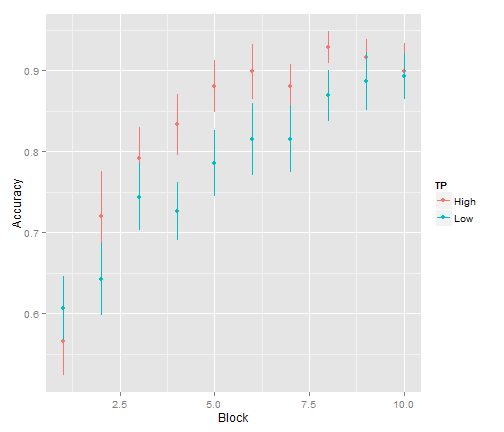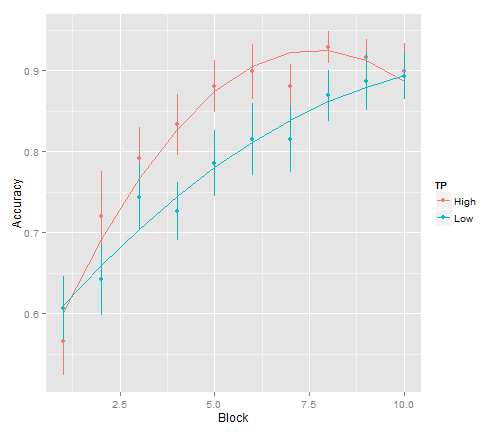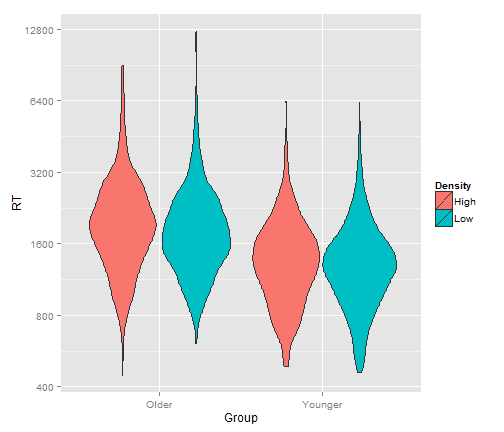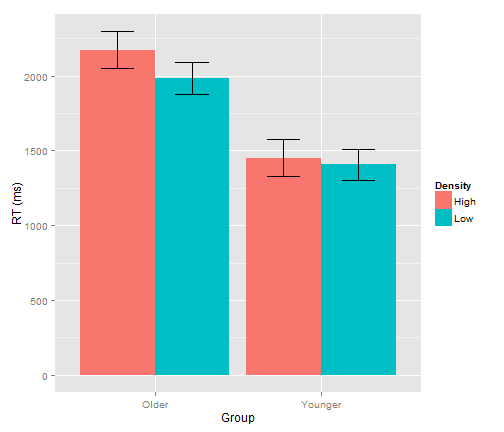# MRRI MLR Demo Examples

### Dan Mirman

These are examples of two different kinds of multilevel regression (MLR) analyses that I presented as part of a very basic introduction to MLR at MRRI in January, 2015. The main point of these examples was just to give people a sense of what running a MLR in R involves, not as full educational examples.

## Example 1: Longitudinal data

Read in data based on a study of effects of transitional probability (TP) on novel word learning (Mirman et al., 2008):

``````##     Subject         TP          Block         Accuracy        Correct
##  Min.   :244.0   High:280   Min.   : 1.0   Min.   :0.000   Min.   :0.00
##  1st Qu.:319.5   Low :280   1st Qu.: 3.0   1st Qu.:0.667   1st Qu.:4.00
##  Median :346.5              Median : 5.5   Median :0.833   Median :5.00
##  Mean   :344.4              Mean   : 5.5   Mean   :0.805   Mean   :4.83
##  3rd Qu.:370.5              3rd Qu.: 8.0   3rd Qu.:1.000   3rd Qu.:6.00
##  Max.   :395.0              Max.   :10.0   Max.   :1.000   Max.   :6.00
##    Incorrect
##  Min.   :0.00
##  1st Qu.:0.00
##  Median :1.00
##  Mean   :1.17
##  3rd Qu.:2.00
##  Max.   :6.00
``````
``````WordLearnEx <- read.delim("http://www.danmirman.org/gca/WordLearnEx.txt")
summary(WordLearnEx)
``````

Here's a graph of those data, made using the `ggplot2` package:

``````library(ggplot2)
ggplot(WordLearnEx, aes(Block, Accuracy, color=TP)) +
stat_summary(fun.data=mean_se, geom="pointrange")
``````We're going to analyze these data using a second-order (quadratic) orthogonal polynomial, so let's build that and add to the data frame

``````#make orthogonal polynomial using the Block values
t <- poly(1:max(WordLearnEx\$Block), 2)
t
``````
``````##                 1           2
##  [1,] -0.49543369  0.52223297
##  [2,] -0.38533732  0.17407766
##  [3,] -0.27524094 -0.08703883
##  [4,] -0.16514456 -0.26111648
##  [5,] -0.05504819 -0.34815531
##  [6,]  0.05504819 -0.34815531
##  [7,]  0.16514456 -0.26111648
##  [8,]  0.27524094 -0.08703883
##  [9,]  0.38533732  0.17407766
## [10,]  0.49543369  0.52223297
## attr(,"degree")
##  1 2
## attr(,"coefs")
## attr(,"coefs")\$alpha
##  5.5 5.5
##
## attr(,"coefs")\$norm2
##    1.0  10.0  82.5 528.0
##
## attr(,"class")
##  "poly"   "matrix"
``````
``````#add it into the data frame
WordLearnEx[, paste("ot", 1:2, sep="")] <- t[WordLearnEx\$Block, 1:2]
#re-check data
summary(WordLearnEx)
``````
``````##     Subject         TP          Block         Accuracy        Correct
##  Min.   :244.0   High:280   Min.   : 1.0   Min.   :0.000   Min.   :0.00
##  1st Qu.:319.5   Low :280   1st Qu.: 3.0   1st Qu.:0.667   1st Qu.:4.00
##  Median :346.5              Median : 5.5   Median :0.833   Median :5.00
##  Mean   :344.4              Mean   : 5.5   Mean   :0.805   Mean   :4.83
##  3rd Qu.:370.5              3rd Qu.: 8.0   3rd Qu.:1.000   3rd Qu.:6.00
##  Max.   :395.0              Max.   :10.0   Max.   :1.000   Max.   :6.00
##    Incorrect         ot1               ot2
##  Min.   :0.00   Min.   :-0.4954   Min.   :-0.34816
##  1st Qu.:0.00   1st Qu.:-0.2752   1st Qu.:-0.26112
##  Median :1.00   Median : 0.0000   Median :-0.08704
##  Mean   :1.17   Mean   : 0.0000   Mean   : 0.00000
##  3rd Qu.:2.00   3rd Qu.: 0.2752   3rd Qu.: 0.17408
##  Max.   :6.00   Max.   : 0.4954   Max.   : 0.52223
``````

We'll start by fitting a base model that has just the time terms (i.e., a model of the overall learning curve), then add the TP condition terms.

``````m.base <- lmer(Accuracy ~ ot1 + ot2 +
(ot1 + ot2 | Subject), data=WordLearnEx, REML=FALSE)
m.0 <- lmer(Accuracy ~ ot1 + ot2 + TP +
(ot1 + ot2 | Subject), data=WordLearnEx, REML=FALSE)
m.1 <- lmer(Accuracy ~ ot1 + ot2 + TP + ot1:TP +
(ot1 + ot2 | Subject), data=WordLearnEx, REML=FALSE)
m.2 <- lmer(Accuracy ~ ot1 + ot2 +
TP + ot1:TP + ot2:TP +
(ot1 + ot2 | Subject), data=WordLearnEx, REML=FALSE)
anova(m.base, m.0, m.1, m.2)
``````
``````## Data: WordLearnEx
## Models:
## m.base: Accuracy ~ ot1 + ot2 + (ot1 + ot2 | Subject)
## m.0: Accuracy ~ ot1 + ot2 + TP + (ot1 + ot2 | Subject)
## m.1: Accuracy ~ ot1 + ot2 + TP + ot1:TP + (ot1 + ot2 | Subject)
## m.2: Accuracy ~ ot1 + ot2 + TP + ot1:TP + ot2:TP + (ot1 + ot2 | Subject)
##        Df     AIC     BIC logLik deviance  Chisq Chi Df Pr(>Chisq)
## m.base 10 -330.77 -287.50 175.39  -350.77
## m.0    11 -330.32 -282.72 176.16  -352.32 1.5506      1    0.21304
## m.1    12 -328.68 -276.75 176.34  -352.68 0.3576      1    0.54984
## m.2    13 -332.63 -276.37 179.32  -358.63 5.9506      1    0.01471 *
## ---
## Signif. codes:  0 '***' 0.001 '**' 0.01 '*' 0.05 '.' 0.1 ' ' 1
``````

Looks like adding the TP effect on the quadratic term significantly improved model fit. In other words, there was a significant effect of TP on the (quadratic) curvature of the learning curves. We can get more detailed output for the full model, including parameter estimates, etc.

``````summary(m.2)
``````
``````## Linear mixed model fit by maximum likelihood  ['lmerMod']
## Formula:
## Accuracy ~ ot1 + ot2 + TP + ot1:TP + ot2:TP + (ot1 + ot2 | Subject)
##    Data: WordLearnEx
##
##      AIC      BIC   logLik deviance df.resid
##   -332.6   -276.4    179.3   -358.6      547
##
## Scaled residuals:
##     Min      1Q  Median      3Q     Max
## -3.6182 -0.5358  0.1263  0.5665  2.6157
##
## Random effects:
##  Groups   Name        Variance Std.Dev. Corr
##  Subject  (Intercept) 0.01076  0.10374
##           ot1         0.01542  0.12419  -0.33
##           ot2         0.00628  0.07925  -0.28 -0.82
##  Residual             0.02456  0.15672
## Number of obs: 560, groups:  Subject, 56
##
## Fixed effects:
##              Estimate Std. Error t value
## (Intercept)  0.831486   0.021727   38.27
## ot1          0.287391   0.037788    7.61
## ot2         -0.167304   0.033188   -5.04
## TPLow       -0.052961   0.030727   -1.72
## ot1:TPLow   -0.001075   0.053441   -0.02
## ot2:TPLow    0.116455   0.046935    2.48
##
## Correlation of Fixed Effects:
##           (Intr) ot1    ot2    TPLow  o1:TPL
## ot1       -0.183
## ot2       -0.114 -0.229
## TPLow     -0.707  0.129  0.081
## ot1:TPLow  0.129 -0.707  0.162 -0.183
## ot2:TPLow  0.081  0.162 -0.707 -0.114 -0.229
``````

and we can plot the model fit

``````ggplot(WordLearnEx, aes(Block, Accuracy, color=TP)) +
stat_summary(fun.data=mean_se, geom="pointrange") +
stat_summary(aes(y=fitted(m.2)), fun.y=mean, geom="line")
``````## Example 2: Crossed random effects of subjects and items

These data are from a pilot study of phonological neighborhood density effects in younger and older adults.

``````##     Subject         Group           Item      Density           RT
##  S103   : 115   Older  :1494   bag    :  30   High:1397   Min.   :  440
##  S122   : 109   Younger:1370   blonde :  30   Low :1467   1st Qu.: 1161
##  S105   : 107                  chain  :  30               Median : 1536
##  S102   : 105                  chair  :  30               Mean   : 1730
##  S108   : 102                  child  :  30               3rd Qu.: 2040
##  S110   : 102                  couch  :  30               Max.   :12753
##  (Other):2224                  (Other):2684
``````
``````density <- read.delim("http://www.danmirman.org/gca/Density.txt")
summary(density)
``````
``````ggplot(density, aes(Group, RT, fill=Density)) + geom_violin() +
scale_y_log10(breaks=c(400, 800, 1600, 3200, 6400, 12800))
``````These are trial-level data (error trials have already been removed). The old-fashioned thing do would be to run separate by-subject and by-item ANOVAs. To do that, we need to compute by-subject and by-item averages (using the `plyr` package)

``````library(plyr)
#by-subject means
density.subj <- ddply(density, .(Subject, Group, Density), summarize, RT = mean(RT))
summary(density.subj)
``````
``````##     Subject       Group    Density         RT
##  S101   : 2   Older  :32   High:30   Min.   :1027
##  S102   : 2   Younger:28   Low :30   1st Qu.:1383
##  S103   : 2                          Median :1603
##  S104   : 2                          Mean   :1743
##  S105   : 2                          3rd Qu.:1960
##  S106   : 2                          Max.   :3601
##  (Other):48
``````
``````#by-item means
density.item <- ddply(density, .(Item, Density, Group), summarize, RT = mean(RT))
summary(density.item)
``````
``````##       Item     Density        Group           RT
##  bag    :  2   High:120   Older  :120   Min.   : 942.2
##  ball   :  2   Low :120   Younger:120   1st Qu.:1366.0
##  band   :  2                            Median :1686.4
##  bean   :  2                            Mean   :1767.4
##  bear   :  2                            3rd Qu.:2040.0
##  bell   :  2                            Max.   :3866.8
##  (Other):228
``````

Now we can run the ANOVAs (repeated measures and mixed within-between ANOVAs are easier to run using the `ez` package)

``````library(ez)
``````
``````## Warning: package 'ez' was built under R version 3.1.2
``````
``````#by-subjects mixed ANOVA with Density as within-subject variable and Group as between-subjects variable
ezANOVA(density.subj, dv=RT, wid=Subject, within=Density, between=Group, type=3)
``````
``````## Warning: Data is unbalanced (unequal N per group). Make sure you specified
## a well-considered value for the type argument to ezANOVA().
``````
``````## \$ANOVA
##          Effect DFn DFd         F            p p<.05         ges
## 2         Group   1  28 17.470709 0.0002590132     * 0.374070220
## 3       Density   1  28 12.455240 0.0014610742     * 0.018425767
## 4 Group:Density   1  28  5.256639 0.0295826013     * 0.007860159
``````
``````#by-items mixed ANOVA with Group as within-item variable and Density as between-items variable
ezANOVA(density.item, dv=RT, wid=Item, within=Group, between=Density, type=3)
``````
``````## \$ANOVA
##          Effect DFn DFd          F            p p<.05         ges
## 2       Density   1 118   2.437651 1.211315e-01       0.014696412
## 3         Group   1 118 223.461729 5.359396e-29     * 0.344870084
## 4 Density:Group   1 118   1.964657 1.636411e-01       0.004606873
``````
• The effect of Group is significant in both.
• The effect of Density is significant by subjects (within-subject effect), but not by items (between-items effect).
• The interaction is significant by subjects but not by items.

Here's how we could analyze these data using MLR with crossed random effects of subjects and items

``````m <- lmer(RT ~ Density*Group + (Density | Subject) + (Group | Item), data=density,
contrasts = list(Density = "contr.sum", Group = "contr.sum"))
``````

There are no p-values due to some ambiguity about how the degrees of freedom should be computed, but for reasonably large data sets, we can just use a normal approximation

``````coefs <- data.frame(coef(summary(m)))
coefs\$p.normal <- 2*(1-pnorm(abs(coefs\$t.value)))
coefs
``````
``````##                   Estimate Std..Error   t.value     p.normal
## (Intercept)     1753.62267   79.49054 22.060772 0.000000e+00
## Density1          58.35157   29.62191  1.969879 4.885224e-02
## Group1           325.28831   75.89407  4.286083 1.818507e-05
## Density1:Group1   36.15172   17.85479  2.024763 4.289168e-02
``````

In the MLR model, each of the effects is statistically significant. We can also use the model to get more reasonable estimates for making a graph (using the `effects` package)

``````library(effects)
``````
``````## Loading required package: lattice
``````
``````ef <- as.data.frame(effect("Density:Group", m))
ef
``````
``````##   Density   Group      fit       se    lower    upper
## 1    High   Older 2173.414 123.9369 1930.400 2416.429
## 2     Low   Older 1984.408 106.8746 1774.849 2193.967
## 3    High Younger 1450.534 124.1431 1207.115 1693.953
## 4     Low Younger 1406.135 104.4298 1201.369 1610.900
``````
``````ggplot(ef, aes(Group, fit, fill=Density)) +
geom_bar(position="dodge", stat="identity") +
geom_errorbar(aes(ymin=fit-se, ymax=fit+se),
width=0.4, position=position_dodge(width=0.9)) +
ylab("RT (ms)")
``````